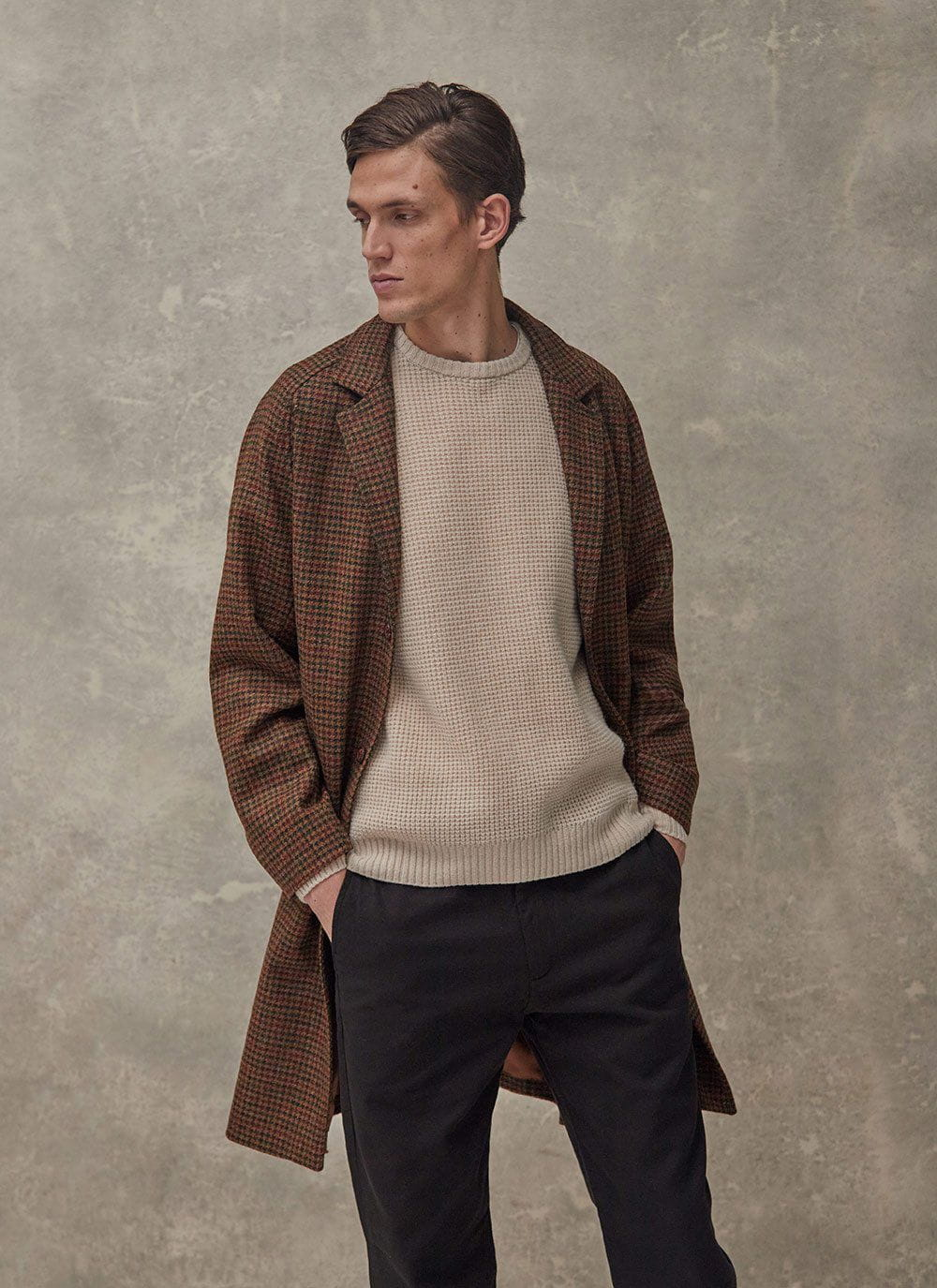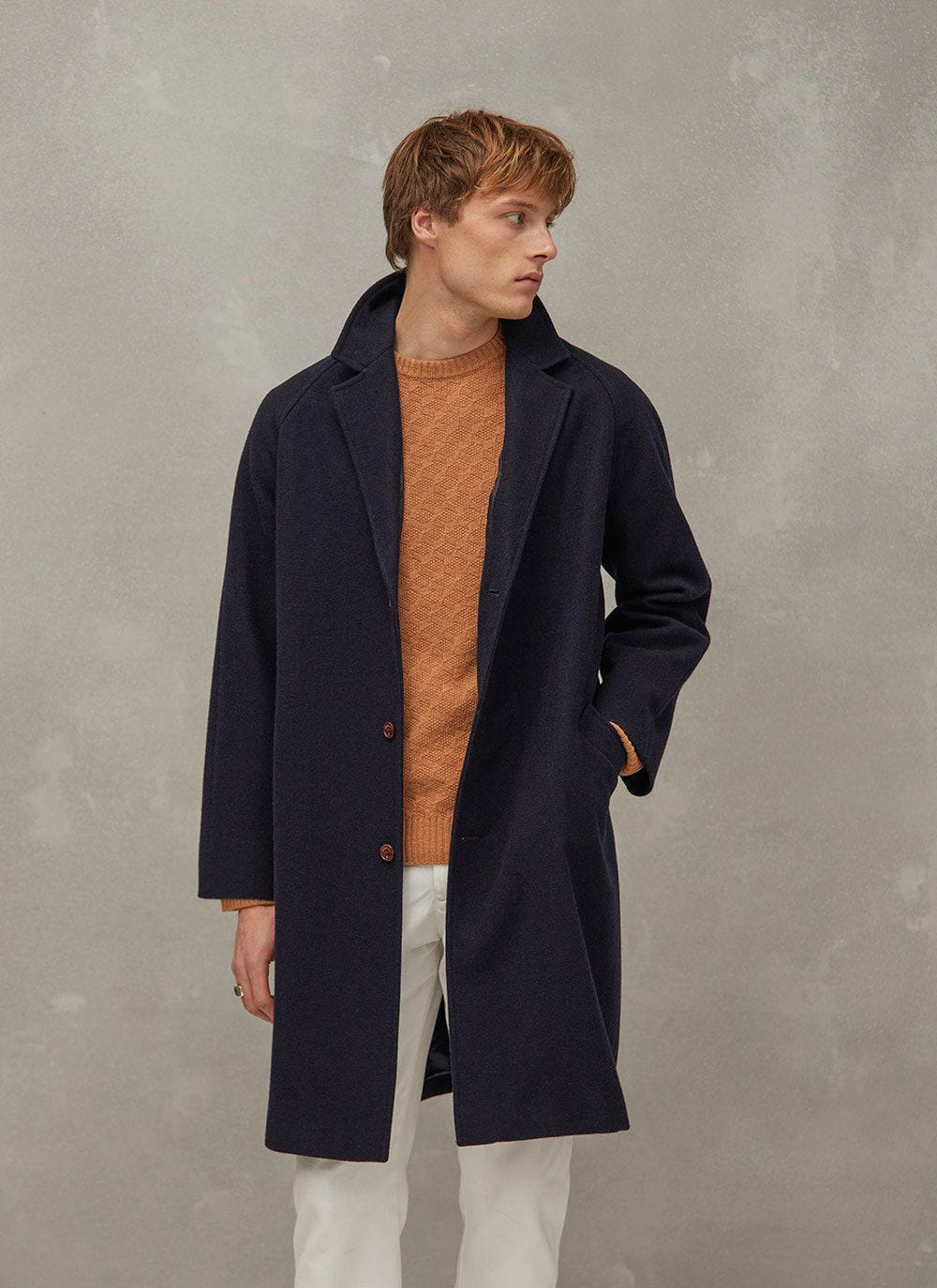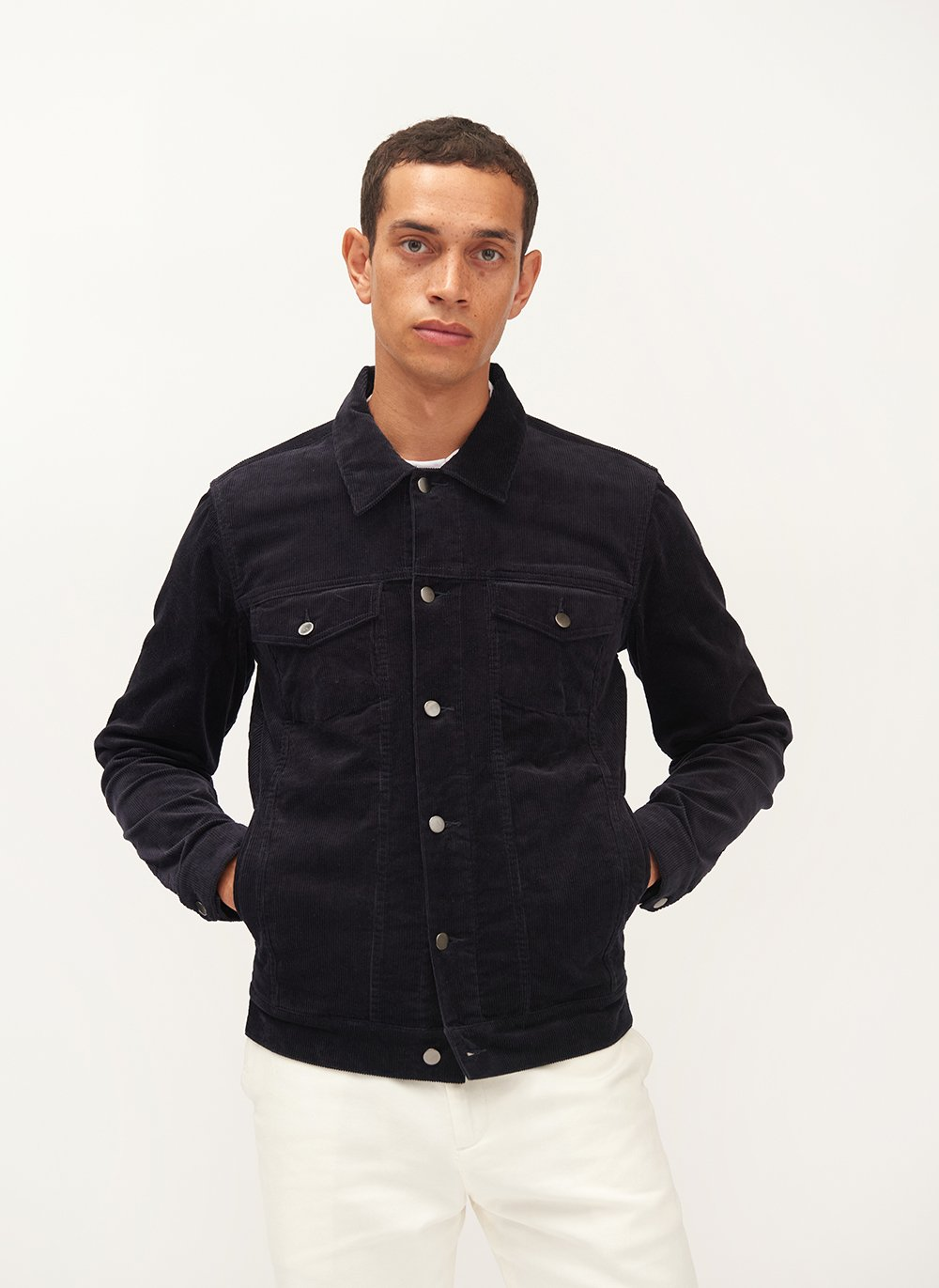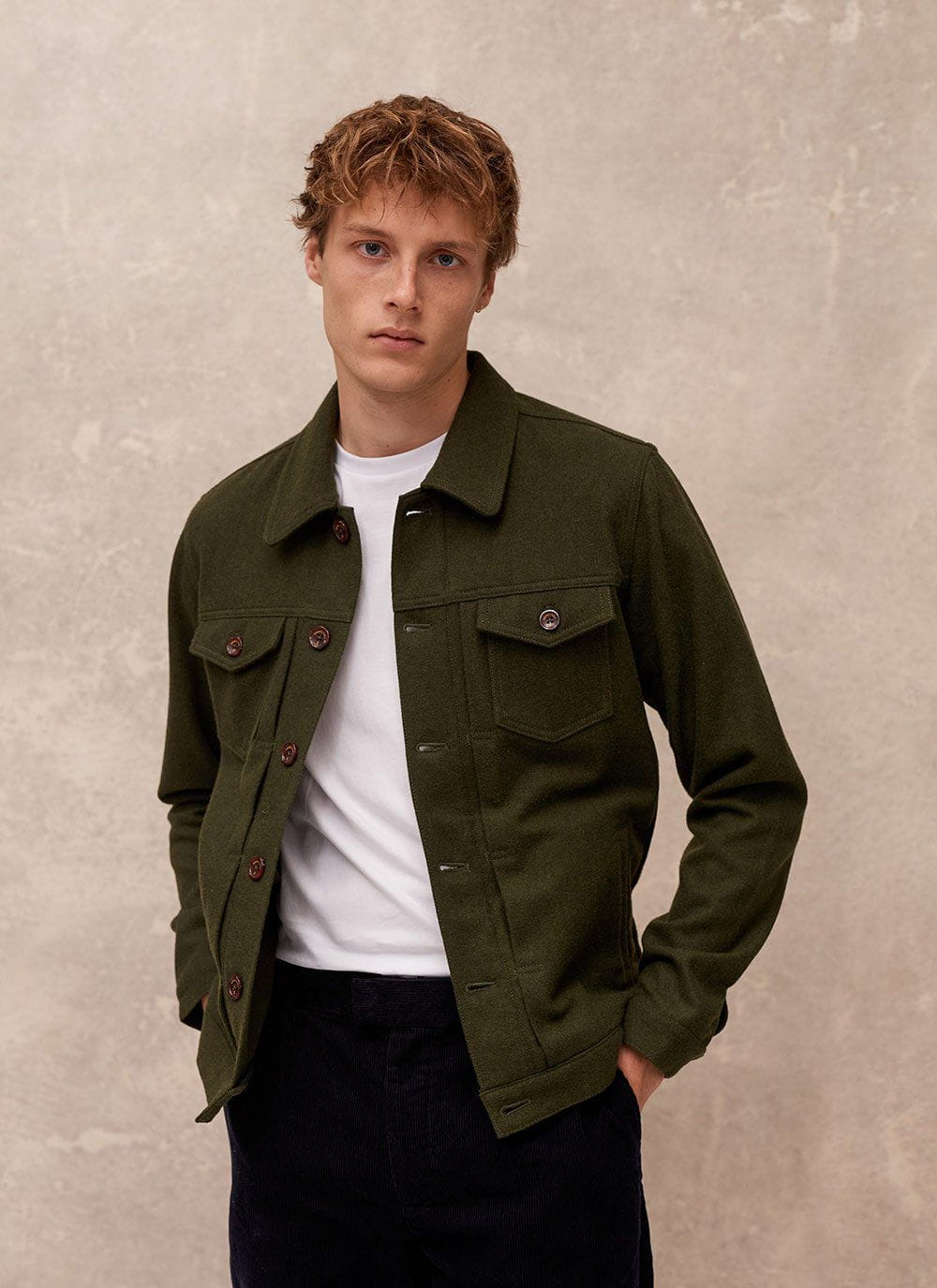Filters
Size

# The Percival Archives - Outerwear

Filters

Selected Filters 

Sold Out• XS
• S
• M
• L
• XL
• XXL
• XXXL
• Select Size
Sold Out• XS
• S
• M
• L
• XL
• XXL
• XXXL
• Select Size
Sold Out• XS
• S
• M
• L
• XL
• XXL
• Select Size• XS
• S
• M
• L
• XL
• XXL
• Select Size
Sold Out• XS
• S
• M
• L
• XL
• XXL
• Select Size• XS
• S
• M
• L
• XL
• XXL
• Select Size• XS
• S
• M
• L
• XL
• XXL
• Select Size
Sold Out• XS
• S
• M
• L
• XL
• XXL
• Select Size• XS
• S
• M
• L
• XL
• XXL
• Select Size• XS
• S
• M
• L
• XL
• XXL
• Select Size• XS
• S
• M
• L
• XL
• XXL
• Select Size
Running Low• XS
• S
• M
• L
• XL
• XXL
• Select Size